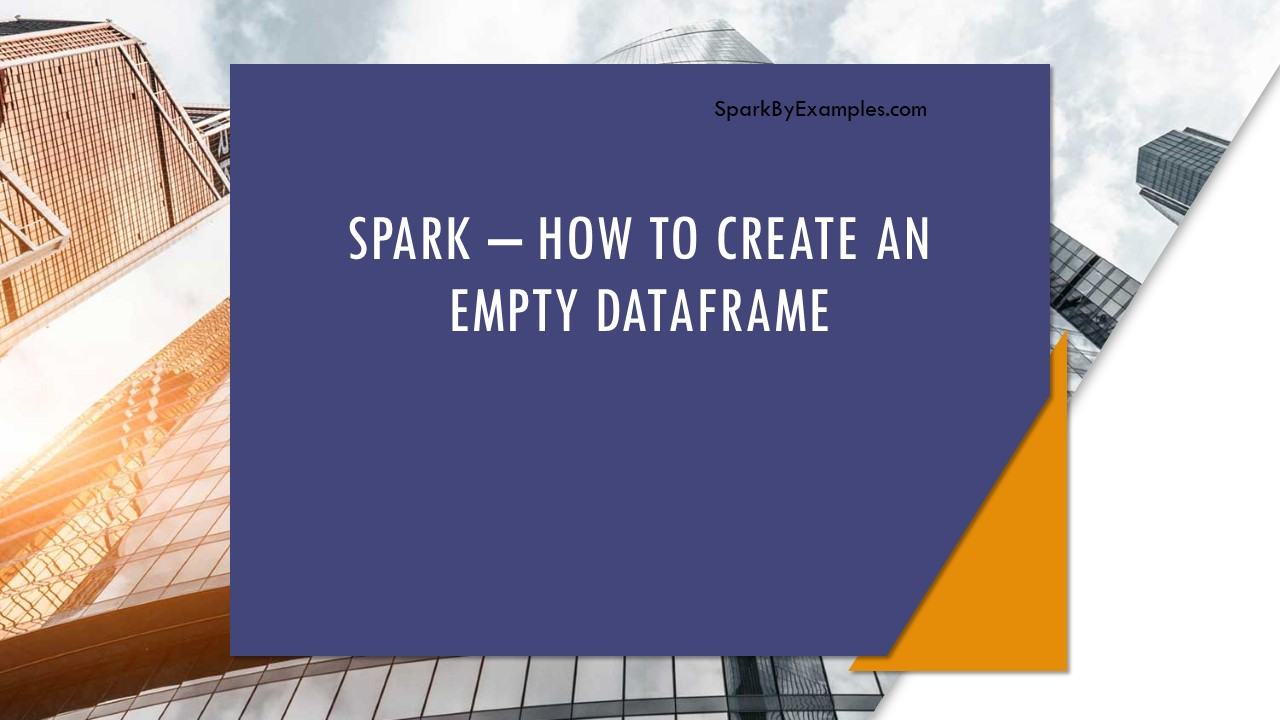# Spark – How to create an empty Dataset?

In this article, I will explain how to create an empty Spark Dataset with or without schema (emptyDataset()) by using several Scala examples. Before we start, I have explained one of the many scenarios where we need to create an empty Dataset.

While working with files in Spark, sometimes we may not receive a file for processing, however, we still need to create an empty Dataset similar (same schema) to the Dataset we create when we receive a file. If we don’t create with the same schema, our operations/transformations on Dataset would fail as we refer to the columns that may not present.

Related: Spark create empty DataFrame

To handle situations similar to these, we always need to create a Dataset with the same schema, which means the same column names and datatypes regardless of the file exists or empty file processing.

First, let’s create a SparkSession and Spark StructType schemas and case class which we will be using throughout our examples.

``````
val spark:SparkSession = SparkSession.builder()
.master("local")
.appName("SparkByExamples.com")
.getOrCreate()

import spark.implicits._

val schema = StructType(
StructField("firstName", StringType, true) ::
StructField("lastName", IntegerType, false) ::
StructField("middleName", IntegerType, false) :: Nil)

val colSeq = Seq("firstName","lastName","middleName")
case class Name(firstName: String, lastName: String, middleName:String)
``````

## 1. emptyDataset() – Create Empty Dataset with zero columns

SparkSession provides an `emptyDataset()` method, which returns the empty Dataset without schema (zero columns), but this is not what we wanted. Below next example shows how to create with schema.

``````
// EmptyDataset() - Create Empty Dataset with zero columns
case class Empty()
val ds0 = spark.emptyDataset[Empty]
ds0.printSchema()

// Outputs following
root
``````

## 2. emptyDataset() – Create Empty Dataset with Schema

Below example create Spark empty Dataset with schema (column names and data types).

``````
val ds1=spark.emptyDataset[Name]
ds1.printSchema()

// Outputs following
root
|-- firstName: string (nullable = true)
|-- lastName: string (nullable = true)
|-- middleName: string (nullable = true)
``````

## 3. createDataset() – Create Empty Dataset with schema

We can create an empty Spark Dataset with schema using `createDataset()` method from SparkSession. The second example below explains how to create an empty RDD first and convert RDD to Dataset.

``````
// CreateDataset() - Create Empty Dataset with schema
val ds2=spark.createDataset(Seq.empty[Name])
ds2.printSchema()
val ds3=spark.createDataset(spark.sparkContext.emptyRDD[Name])
ds3.printSchema()

//These both Outputs following
root
|-- firstName: string (nullable = true)
|-- lastName: string (nullable = true)
|-- middleName: string (nullable = true)
``````

## 4. createDataset() – Create Empty Dataset with default column names

``````
// CreateDataset() - Create Empty Dataset with default column names
val ds4=spark.createDataset(Seq.empty[(String,String,String)])
ds4.printSchema()
// Outputs following
root
|-- _1: string (nullable = true)
|-- _2: string (nullable = true)
|-- _3: string (nullable = true)
``````

## 5. Using implicit encoder

Let’s see another way, which uses implicit encoders.

``````
// Using implicit encoder
val ds5 = Seq.empty[(String,String,String)].toDS()
ds5.printSchema()
// Outputs following
root
|-- _1: string (nullable = true)
|-- _2: string (nullable = true)
|-- _3: string (nullable = true)
``````

## 6. Using case class

We can also create empty Dataset with the schema we wanted from scala case class.

``````
// Using case class
val ds6 = Seq.empty[Name].toDS()
ds6.printSchema()

// Outputs following
root
|-- firstName: string (nullable = true)
|-- lastName: string (nullable = true)
|-- middleName: string (nullable = true)
``````

Happy Learning !!

### Naveen (NNK)

Naveen (NNK) is a Data Engineer with 20+ years of experience in transforming data into actionable insights. Over the years, He has honed his expertise in designing, implementing, and maintaining data pipelines with frameworks like Apache Spark, PySpark, Pandas, R, Hive and Machine Learning. Naveen journey in the field of data engineering has been a continuous learning, innovation, and a strong commitment to data integrity. In this blog, he shares his experiences with the data as he come across. Follow Naveen @ @ LinkedIn

### This Post Has 2 Comments

1.PANKAJ

Lovely discussions/examples, there are some syntax error at the time of execution in python 3.8 on Windows 10 environment. Numpy runtime error,
Also
val spark:SparkSession = SparkSession.builder()

SyntaxError: invalid syntax
RuntimeError: The current Numpy installation (‘c:\\users\\ppchanda\\appdata\\local\\programs\\python\\python38\\lib\\site-packages\\numpy\\__init__.py’) fails to pass a sanity check due to a bug in the windows runtime

1.NNK

Hi Pankaj, seems this issue is related to your environment. Examples specified here were tested in my development environment. There could be some typo’s here and there but not the issues you are mentioned.
Wondering if you able to resolve the issue? if yes, please comment on how you overcome this.